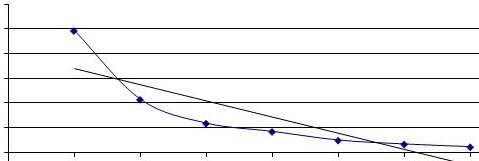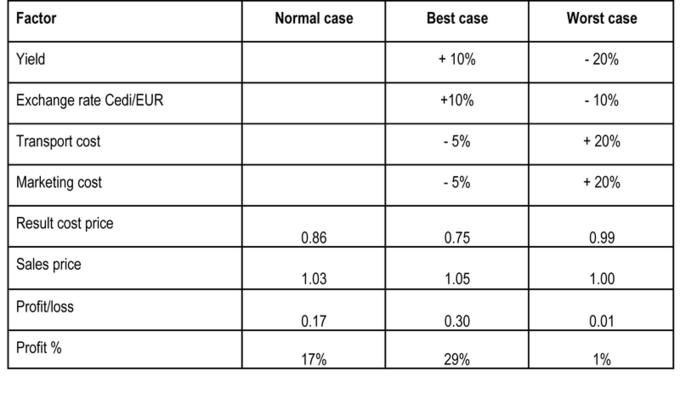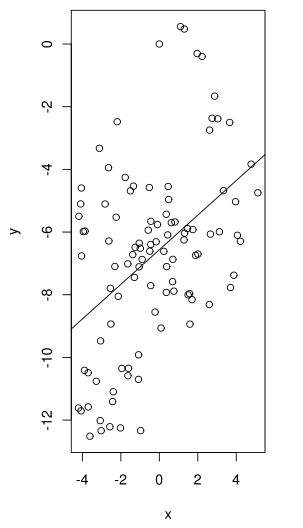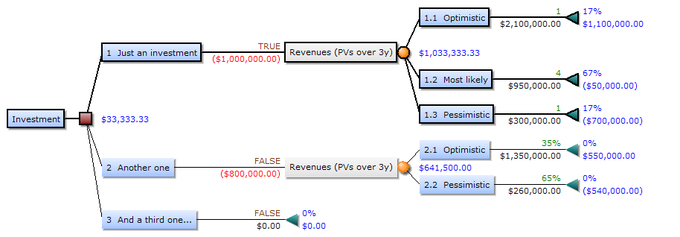## Sensitivity Analysis

Sensitivity analysis determines how much a change in an input will affect the output.

### Learning Objectives

Describe how sensitivity analysis is used to make investment decisions

### Key Takeaways

#### Key Points

• Since variations from the base assumptions are expected, businessmen and women want to know how much their output (eg., revenue) will be affected by the variations.
• Sensitivity analysis helps find the optimal levels for inputs (eg., raw material prices, number of employees, sales price).
• Sensitivity analysis is a statistical tool based on seeing how inputs and parameters affect outputs. Generally, each input is changed one at a time to see how it affects output. However, this does not account for interconnectedness between inputs; they may not be independent variables.

#### Key Terms

• parameter: A variable kept constant during an experiment, calculation, or similar.
• sensitivity analysis: the study of how the uncertainty in the output of a mathematical model or system can be apportioned to different sources of uncertainty in its inputs

Capital budgeting is, by definition, forward looking. When dealing with expected resources and demands, uncertainty is a major factor. Sensitivity analysis is a statistical tool that determines how consequential deviations from the expected value occur. Sensitivity Analysis deals with finding out the amount by which we can change the input data for the output of our linear programming model to remain comparatively unchanged. This helps us in determining the sensitivity of the data we supply for the problem. It also helps to determine the optimal levels of each input.

Sensitivity analysis can be useful for a number of reasons, including:

• Support decision making or the development of recommendations for decision makers (e.g., testing the robustness of a result).
• Enhance communication from modelers to decision makers (e.g., by making recommendations more credible, understandable, compelling or persuasive).
• Increase understanding or quantification of the system (e.g., understanding relationships between input and output variables).
• Model development (e.g., searching for errors in the model).

In order to conduct a sensitivity analysis, all of the inputs and parameters are connected via an algorithm to produce the output. For example, a model of the inputs and parameters for a company interest in creating a new product may include information about expected availability of raw material, inflation rates, and number of employees working in R&D. The output would be the profit generated by the new product. The sensitivity analysis entails changing each variable and seeing how that changes the output. Generally, only one variable is changed at once, with all of the others fixed at their base value. This makes it easy to see how much a variable affects the output. However, not all of the inputs may be independent so changing inputs one at a time does not account for interaction between the inputs.Sensitivity of a Variable: Sensitivity analysis determines how much an output is expected to change due to changes in a variable or parameter. In this case, the output (y-axis) decreases exponentially with an increase in the input (x-axis). This is mapped out for each input.

## Scenario Analysis

Scenario analysis is a process of analyzing decisions by considering alternative possible outcomes.

### Learning Objectives

Explain scenario analysis is used by investors

### Key Takeaways

#### Key Points

• Scenario analysis is designed to see the consequences of an action under different sets of factors. For example, it shows how an investment ‘s NPV would differ under high and low inflation.
• Scenarios should be feasible enough to provide an accurate picture of the outcomes. A “good” scenario for an investor should not include winning the lottery because, though good, it is neither probable nor realistic for analyzing possible results.
• Many scenario analyses use 3 scenarios: base case, worst case and best case. However, the number and conditions of the scenarios in each analysis can vary.

#### Key Terms

• scenario: A set of factors that can affect the consequences of an action. The environment.
• analysis: A process of dismantling or separating into constituent elements in order to study the nature, function, or meaning.
• scenario analysis: a process of analyzing possible future events by considering alternative possible outcomes

### Scenario Analysis

Scenario analysis is a strategic process of analyzing decisions by considering alternative possible outcomes (sometimes called “alternative worlds”). It is not a predictive mechanism, but rather an analytic tool to manage uncertainty today..Scenario Analysis: This scenario analysis shows how changes in factors like yield and transport cost can affect profits.

For example, a firm might use scenario analysis to determine the net present value (NPV) of a potential investment under high and low inflation scenarios.

In another example, a bank might attempt to forecast several possible scenarios for the economy (e.g. rapid vs. moderate vs. slow growth) or it might try to forecast financial market returns (for bonds, stocks and cash) in each of those scenarios. Perhaps, it might also consider sub-sets of each of the possibilities. It might further seek to determine correlations and assign probabilities to the scenarios (and sub-sets if any). By analyzing these various scenarios, the bank will be in a better position to consider how best to allocate its assets.

Many scenario analyses use three different scenarios: base case, worst case and best case. The base case is the expected scenario: if all things proceed normally, this is what the expected outcome will be. The worst and best cases are obviously scenarios with less and more favorable conditions, but they are still confined by a sense of feasibility. For example, an investor creating the worst case scenario would not be well served to have it include a meteor strike that destroys the company. While clearly a bad scenario, it is not realistic enough to be helpful.

The purpose of scenario analysis is not to identify the exact conditions of each scenario; it just needs to approximate them to provide a plausible idea of what might happen.

## Monte Carlo Simulation

Monte Carlo simulation uses statistical data to figure out the average outcome of a scenario based on multiple, complex factors.

### Learning Objectives

Describe how Monte Carlo simulations are used

### Key Takeaways

#### Key Points

• The statistical distribution is estimated for each input (eg. inflation rate, market risk ). Then, simulations are run to see how constantly changing inputs (based on their distribution) affect the output. The outputs are averaged to find the estimated output.
• Monte Carlo simulations are great for when there are multiple inputs that can all change and may be unrelated.
• Monte Carlo simulations can be applied in many areas of business, such as bond pricing, but are especially useful when estimating the “base case” is difficult to do by hand.

#### Key Terms

• distribution: A probability distribution; the set of relative likelihoods that a variable will have a value in a given interval.Monte Carlo Simulation: By running many simulations based on the probability or distribution of an input (x), the analyst can see the average output (y). This is done for multiple inputs at once to find out how they affect the output.

In order to account of complex, interconnected factors, all of which may affect financial outcomes, companies turn to statistical methods. The Monte Carlo method solves a problem by directly simulating the underlying process and then calculating the average result of the process. It simulates the various sources of uncertainty (eg. inflation, default risk, market changes, etc.) that affect the value of the instrument, portfolio, or investment in question, and calculates a representative value given these possible values of the underlying inputs. In essence, the Monte Carlo method is designed to find out what happens to the outcome on average when there are changes in the inputs.

Each potential factor is assigned a probability or statistical distribution. For example, the investor may estimate the probability of default on a bond as 20%. That means that 20% of the time, he will not earn back his principal. The investor may also estimate that the inflation rate is normally distributed around a mean of 3% and standard deviation of 0.5%.

The investor estimates the probability or distribution of every factor that could change the result of the investment. Then, he essentially uses the distributions to run many many simulations of all the inputs to see how they affect the output and then finds the average output.

For example, for bonds and bond options, under each possible evolution of interest rates the investor observes a different yield curve and a different resultant bond price. To determine the bond value, these bond prices are then averaged. To value the bond option, as for equity options, the corresponding exercise values are averaged and present valued. By determining the average, the investor can figure out what the expected value is.

The advantage of the Monte Carlo method is that it is able to handle multiple moving, and possible related, inputs. As the number of factors increases, it becomes harder to figure out the “base case. ” Statistical analysis through Monte Carlo simulations is great at handling problems with multiple, inter-related, and uncertain factors.

## Decision Trees

A decision tree is a decision support tool that uses a tree-like graph or model of decisions and their possible consequences.

### Learning Objectives

Describe how to set up a decision tree

### Key Takeaways

#### Key Points

• Decision trees look similar to flow charts, except they are designed to pick the most optimal strategy.
• There are three types of nodes on a decision tree: decision nodes, chance nodes, and end nodes.
• Decision trees are solved from top to bottom in order to determine the most optimal strategy. Sometimes, there may not be an obvious optimal strategy, but the tree is still useful for mapping out the options faced and their costs/ benefits.

#### Key Terms

• node: A vertex point on a decision tree. Other branches may extend from it; if none do, it is an end node.

A decision tree is a decision support tool that uses a tree-like graph or model of decisions and their possible consequences, including chance event outcomes, resource costs, and utility. They help to identify the strategy that is most likely to reach the declared goal. For an investor, this may be used to help determine which bond to buy in order to get the highest expected return with only a certain amount of risk. Decision trees are set up much like an organizational flow chart.Decision Tree: This decision tree highlights the outcomes of different investing strategies. Next to the terminal nodes is blue text with the yield and gain.

Unlike a flow chart, a decision tree consists of three types of nodes:

1. Decision nodes – commonly represented by squares – the user gets to decide which branch to take.
2. Chance nodes – represented by circles – the branch taken is determined by probabilities.
3. End nodes – represented by triangles – there are no more branches extending from the node, and the final value of the strategy is listed.

In order to set up and use a decision tree, the user must first list each decision point. For example, in, the investor first listed the number of investments that could be made (1, 2, or 3) and then the options for the likely payoffs (optimistic, most likely, etc.). Each end node was given a value. Working from left to right (or top to bottom), the investor determined that making one investment has the highest expected value ($641,500 vs.$0). Therefore, the investor will definitely choose to make one investment. Then, to determine the payoff, the probabilities and yields are listed. This is a chance node, so the investor doesn’t know how much he will earn from the investment, but does know his options.

Decision trees can become very complex, so determining the correct strategy becomes more difficult. Decision trees are always solved from the top to the bottom (or left to right) and is just a process of picking the most optimal path or seeing the likely payoffs.

There may not be an idea strategy shown on the decision tree. In that case, the decision tree is still useful as a way to map out all of the possible strategies with their costs and benefits.